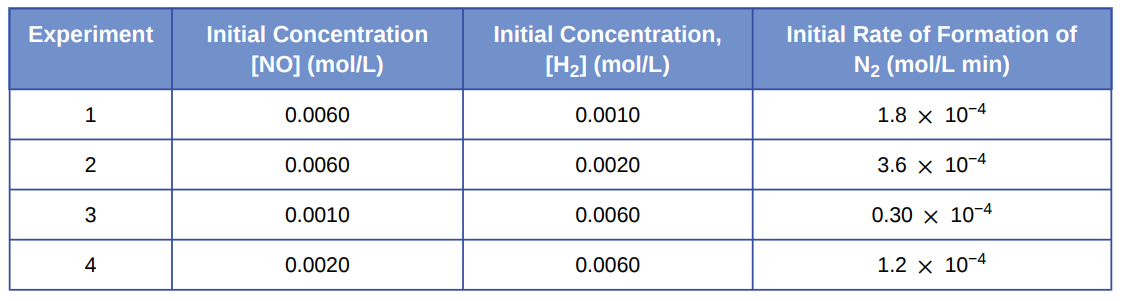# Problem: Experiments were conducted to study the rate of the reaction represented by this equation. 2 NO(g) + 2 H2(g) ⟶ N2(g) + 2 H2O(g) Initial concentrations and rates of reaction are given here.Consider the following questions: (a) Determine the order for each of the reactants, NO and H2, from the data given and show your reasoning.

###### FREE Expert Solution
94% (181 ratings)
###### Problem Details

Experiments were conducted to study the rate of the reaction represented by this equation.
2 NO(g) + 2 H2(g) ⟶ N2(g) + 2 H2O(g)
Initial concentrations and rates of reaction are given here.Consider the following questions:

(a) Determine the order for each of the reactants, NO and H2, from the data given and show your reasoning.

Frequently Asked Questions

What scientific concept do you need to know in order to solve this problem?

Our tutors have indicated that to solve this problem you will need to apply the Rate Law concept. You can view video lessons to learn Rate Law. Or if you need more Rate Law practice, you can also practice Rate Law practice problems.

What textbook is this problem found in?

Our data indicates that this problem or a close variation was asked in Chemistry - OpenStax 2015th Edition. You can also practice Chemistry - OpenStax 2015th Edition practice problems.